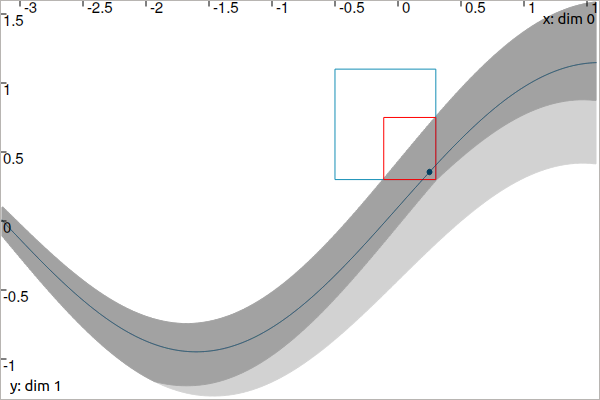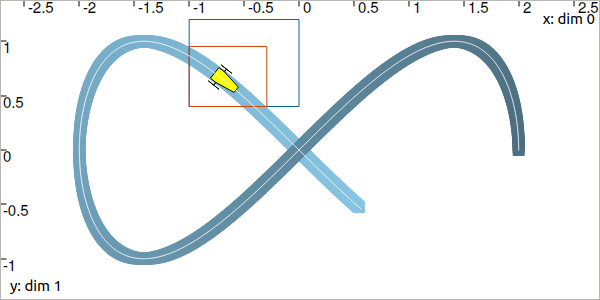# CtcEval: $$y_i=x(t_i)$$¶

$$y_i=x(t_i)$$ is a constraint that links a value $$y_i$$ to the evaluation of the trajectory $$x(\cdot)$$ at time $$t_i$$. A dedicated contractor $$\mathcal{C}_{\textrm{eval}}$$ implements this constraint. One of its applications is the correction of the positions of a robot based on observations.

## Definition¶

Important

$\begin{split}\left.\begin{array}{r}y_i=x(t_i)\\\dot{x}(\cdot)=v(\cdot)\end{array}\right\} \longrightarrow \mathcal{C}_{\textrm{eval}}\big([t_i],[y_i],[x](\cdot),[v](\cdot)\big)\end{split}$
ctc.eval.contract(ti, yi, x, v)

ctc::eval.contract(ti, yi, x, v);


Prerequisite

The tubes $$[x](\cdot)$$ and $$[v](\cdot)$$ must share:

• the same slicing (same sampling of time)

• the same t-domain $$[t_0,t_f]$$

• the same dimension of $$[y_i]$$ in the vector case

## 1d theoretical presentation¶

The $$\mathcal{C}_{\textrm{eval}}$$ contractor aims at intersecting a tube $$[x](\cdot)$$ by the envelope of all trajectories compliant with the bounded observation $$[t_i]\times[y_i]$$. In other words, $$[x](\cdot)$$ will be contracted by the tube of all $$x(\cdot)\in[x](\cdot)$$ going through the box $$[t_i]\times[y_i]$$, as shown in Fig. 22. Some trajectories may partially cross the box at some point over $$[t_i]$$: the contractor must take into account the feasible propagations during the intersection process. To this end, the knowledge of the derivative $$\dot{x}(\cdot)$$ is required to depict the evolution of $$x(\cdot)$$ over $$[t_i]$$.

The contractor is proposed in the most generic way; the derivative $$\dot{x}(\cdot)$$ is also bounded within a tube denoted $$[v](\cdot)$$, thus allowing the $$[x](\cdot)$$ contraction even if the derivative signal is uncertain.Fig. 22 Observation on a tube $$[x](\cdot)$$. A given measurement $$\mathbf{m}\in\mathbb{R}^{2}$$, pictured by a black dot, is known to belong to the blue box $$[t_i]\times[y_i]$$. The sets are contracted by means of $$\mathcal{C}_{\textrm{eval}}$$; the contracted part of the tube is depicted in light gray. Meanwhile, the bounded observation itself is contracted to $$[t_i']\times[y_i']$$ with $$[t_i']\subseteq[t_i]$$ and $$[z_i']\subseteq[z_i]$$. This is illustrated by the red box. The dark line is an example of a compliant trajectory. The derivative $$\dot{x}(\cdot)$$, not represented here, is also enclosed within a tube.

The code leading to the contraction presented in this figure is:

dt = 0.01
tdomain = Interval(-math.pi, math.pi/2)

v = Tube(tdomain, dt, TFunction("cos(t)+[-0.1,0.1]")) # uncertain derivative (not displayed)
x = v.primitive() + Interval(-0.1, 0.1) # x is the primitive of v

# Bounded observation
ti = Interval(-0.5,0.3)
yi = Interval(0.3,1.1)

# Contraction
ctc_eval = CtcEval()
ctc_eval.contract(ti, yi, x, v)
# Note that we could use directly ctc.eval.contract(ti, yi, x, v)

double dt = 0.01;
Interval tdomain(-M_PI, M_PI/2.);

Tube v(tdomain, dt, TFunction("cos(t)+[-0.1,0.1]")); // uncertain derivative (not displayed)
Tube x = v.primitive() + Interval(-0.1, 0.1); // x is the primitive of v

// Bounded observation
Interval ti(-0.5,0.3);
Interval yi(0.3,1.1);

// Contraction
CtcEval ctc_eval;
ctc_eval.contract(ti, yi, x, v);
// Note that we could use directly ctc::eval.contract(ti, yi, x, v)


Restrict the temporal propagation (save computation time)

$$\mathcal{C}_{\textrm{eval}}$$ may contract the tube $$[x](\cdot)$$ and in this case, the contraction will be temporally propagated forward/backward in time from the $$[t_i]$$ t-domain. In Fig. 22, we can see that the contraction occurs over $$[-1.9,t_f]$$. The .enable_time_propag(false) method can be used to limit the contraction to the $$[t_i]$$ t-domain only. This is useful when dealing with several observations $$[t_i]\times[z_i]$$ on the same tube: it becomes faster to first perform all the local contractions over each $$[t_i]$$ and then smooth the tube only once with, for instance, the $$\mathcal{C}_{\frac{d}{dt}}$$ contractor presented before.

For instance, we now consider three constraints on the tube:

ti = [Interval(-0.5,0.3), Interval(-0.6,0.8), Interval(-2.3,-2.2)]
yi = [Interval(0.3,1.1), Interval(-0.5,-0.4), Interval(-0.8,-0.7)]

Interval ti, yi;
ti = Interval(-0.5,0.3); yi = Interval(0.3,1.1);
ti = Interval(-0.6,0.8); yi = Interval(-0.5,-0.4);
ti = Interval(-2.3,-2.2); yi = Interval(-0.8,-0.7);


Then we use the contractor configured for the limited contraction:

ctc_eval.enable_time_propag(False)

for i in range (0,3):
ctc_eval.contract(ti[i], yi[i], x, v)

ctc.deriv.contract(x, v) # for smoothing the tube

for i in range (0,3): # for contracting the [ti]×[yi] boxes
ctc_eval.contract(ti[i], yi[i], x, v)

ctc_eval.enable_time_propag(false);

for(int i = 0 ; i < 3 ; i++)
ctc_eval.contract(ti[i], yi[i], x, v);

ctc::deriv.contract(x, v); // for smoothing the tube

for(int i = 0 ; i < 3 ; i++) // for contracting the [ti]×[yi] boxes
ctc_eval.contract(ti[i], yi[i], x, v);


The following animation presents the results before and after the $$\mathcal{C}_{\frac{d}{dt}}$$ contraction:Fixed point propagation

When dealing with several constraints on the same tube, a single application of $$\mathcal{C}_{\textrm{eval}}$$ for each $$[t_i]\times[y_i]$$ may not provide optimal results. Indeed, $$\mathcal{C}_{\textrm{eval}}$$ propagates an evaluation along the whole domain of $$[x](\cdot)$$ which may lead to new possible contractions. It is preferable to use an iterative method that applies all contractors indefinitely until they become ineffective on $$[x](\cdot)$$ and the $$[t_i]\times[y_i]$$’s:

The CN chapter for constraint propagation.

## 2d localization example¶

Contracting the tube

Let us come back to the Lissajous example of the previous page.

Assume now that we have no knowledge on $$[\mathbf{x}](\cdot)$$, except that the feasible trajectories go through the box $$[\mathbf{b}]=[-0.73,-0.69]\times[0.64,0.68]$$ at some time $$t\in[4.3,4.4]$$.

The tube is contracted over $$[t_0,t_f]$$ with its uncertain derivative $$[\mathbf{v}](\cdot)$$ given by:

$\begin{split}[\mathbf{v}](t) = \left(\begin{array}{c}-2\sin(t)\\2\cos(2t)\end{array}\right)+\left(\begin{array}{c} [-0.01,0.01]\\ [-0.01,0.01]\end{array}\right)\end{split}$
dt = 0.01
tdomain = Interval(0,5)
# No initial knowledge on [x](·)
x = TubeVector(tdomain, dt, 2) # initialization with [-∞,∞]×[-∞,∞]

# New values for the temporal evaluation of [x](·)
t = Interval(4.3,4.4)
b = IntervalVector([[-0.73,-0.69],[0.64,0.68]])

# Uncertain derivative of [x](·)
v = TubeVector(tdomain, dt, TFunction("(-2*sin(t) ; 2*cos(2*t))"))
v.inflate(0.01)

# Contraction
ctc_eval = CtcEval()
ctc_eval.contract(t, b, x, v)
# Note that in this case, no contraction is performed on [t] and [b]
# Note also that we could use directly ctc.eval.contract(t, b, x, v)

double dt = 0.01;
Interval tdomain(0.,5.);
// No initial knowledge on [x](·)
TubeVector x(tdomain, dt, 2); // initialization with [-∞,∞]×[-∞,∞]

// New values for the temporal evaluation of [x](·)
Interval t(4.3,4.4);
IntervalVector b({{-0.73,-0.69},{0.64,0.68}});

// Uncertain derivative of [x](·)
TubeVector v(tdomain, dt, TFunction("(-2*sin(t) ; 2*cos(2*t))"));
v.inflate(0.01);

// Contraction
CtcEval ctc_eval;
ctc_eval.contract(t, b, x, v);
// Note that in this case, no contraction is performed on [t] and [b]
// Note also that we could use directly ctc::eval.contract(t, b, x, v)


The obtained tube is blue painted on the figure, the contraction to keep the trajectories going through $$[\mathbf{b}]$$ (red box) over $$[t]=[4.3,4.4]$$ is propagated over the whole t-domain:Contracting the evaluation box

Assume now that we know the actual trajectory to be bounded within the tube:

$\begin{split}[\mathbf{x}](t) = \left(\begin{array}{c}2\cos(t)\\\sin(2t)\end{array}\right)+\left(\begin{array}{c} [-0.05,0.05]\\ [-0.05,0.05]\end{array}\right)\end{split}$
x = TubeVector(tdomain, dt, TFunction("(2*cos(t) ; sin(2*t))"))
x.inflate(0.05)

TubeVector x(tdomain, dt, TFunction("(2*cos(t) ; sin(2*t))"));
x.inflate(0.05);


The tube is blue painted on the figure:The yellow robot depicts an unknown position $$\mathbf{x}$$ in the box $$[-1,0]\times[0.4,1.2]$$ at an unknown $$t\in[t_0,t_f]$$. The $$\mathcal{C}_{\textrm{eval}}$$ can be used to evaluate the position time and reduce the uncertainty on the possible positions.

t = Interval(0,oo) # new initialization
b = IntervalVector([[-1,0],[0.4,1.2]]) # (blue box on the figure)

ctc_eval.contract(t, b, x)

# [t] estimated to [4.15, 4.54]
# [b] contracted to ([-1, -0.29] ; [0.4, 0.95])  (red on the figure)

t = Interval(); // new initialization
b = {{-1.,0.},{0.4,1.2}}; // (blue box on the figure)

ctc_eval.contract(t, b, x);

// [t] estimated to [4.15, 4.54]
// [b] contracted to ([-1, -0.29] ; [0.4, 0.95])  (red on the figure)